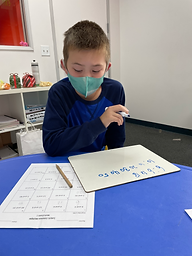Ms. Lily

Target 1​

Lesson Type:

Review

Number Operation

:

Integer Composition

Recognize a whole number is a multiple of each of its factors.

1:

Determine whether a given whole number in the range of 1 to 100 is a multiple of a given one-digit number.

2:

Recognize patterns in multiples, setting the foundation for developing computational fluency in multiplication.

4th

Vocabulary:

Factors, Multiplication

Activities:

• Students played a board game called Multiples Roll and Solve, where they rolled dice and found multiples of it on the board.
• Students played a Factors & Multiples chain game.Home Exploration

Guiding Questions:Absent Students:

Target 2

:

1:

Define Least Common Multiple (LCM).

2:

Find all the factor pairs of a number.

3:

Understand factor pairs as two whole numbers that multiple together to get one product.

4:

List the LCM for two whole numbers.

4th

Vocabulary:

Least Common Factor (LCM), Factors, Multiples

Activities:

• Students found the Least Common Multiple (LCM) for different pairs of numbers.
• Students played a game called Factor Freeze, where they rolled factors and found the product.Home Exploration

Guiding Questions:Target 3

:

1:

Use the given information in a problem to identify the correct answer.

2:

Use the given information to determine which is not the correct answer.

3:

Think outside of the box to solve problems.

4:

Follow given rules to solve problems.

4th

Vocabulary:

Clues, Hints

Activities:

• Students solved various Christmas-themed logic puzzles.Home Exploration